# Learning a fair loss function in pytorch

Most of the time when we are talking about deep learning, we are discussing really complicated architectures – essentially complicated sets of (mostly linear) equations. A second innovation in the application of deep learning though is the use of back propagation to fit under-determined systems. Basically you can feed these systems fairly complicated loss functions, and they will chug along with no problem. See a prior blog post of mine of creating simple regression weights for example.

Recently in the NIJ challenge, I used pytorch and the non-linear fairness function defined by NIJ. In the end me and Gio used an XGBoost model, because the data actually do not have very large differences in false positive rates between racial groups. I figured I would share my example here though for illustration of using pytorch to learn a complicated loss function that has fairness constraints. And here is a link to the data and code to follow along if you want.

First, in text math the NIJ fairness function was calculated as `(1 - BS)*(1 - FP_diff)`, where BS is the Brier Score and FP_diff is the absolute difference in false positive rates between the two groups. My pytorch code to create this loss function looks like this (see the `pytorch_mods.py` file):

``````# brier score loss function with fairness constraint
def brier_fair(pred,obs,minority,thresh=0.5):
bs = ((pred - obs)**2).mean()
over = 1*(pred > thresh)
majority = 1*(minority == 0)
fp = 1*over*(obs == 0)
min_tot = (over*minority).sum().clamp(1)
maj_tot = (over*majority).sum().clamp(1)
min_fp = (fp*minority).sum()
maj_fp = (fp*majority).sum()
min_rate = min_fp/min_tot
maj_rate = maj_fp/maj_tot
diff_rate = torch.abs(min_rate - maj_rate)
fin_score = (1 - bs)*(1 - diff_rate)
return -fin_score``````

I have my functions all stashed in different py files. But here is an example of loading up all my functions, and fitting my pytorch model to the training recidivism data. Here I set the threshold to 25% instead of 50% like the NIJ competition. Overall the model is very similar to a linear regression model.

``````import data_prep as dp
import fairness_funcs as ff
import pytorch_mods as pt

# Get the train/test data
train, test = dp.get_y1_data()
y_var = 'Recidivism_Arrest_Year1'
min_var = 'Race' # 0 is White, 1 is Black
x_vars = list(train)
x_vars.remove(y_var)

# Model learning fair loss function
m2 = pt.pytorchLogit(loss='brier_fair',activate='ident',
minority=min_var,threshold=0.25)
m2.fit(train[x_vars],train[y_var])``````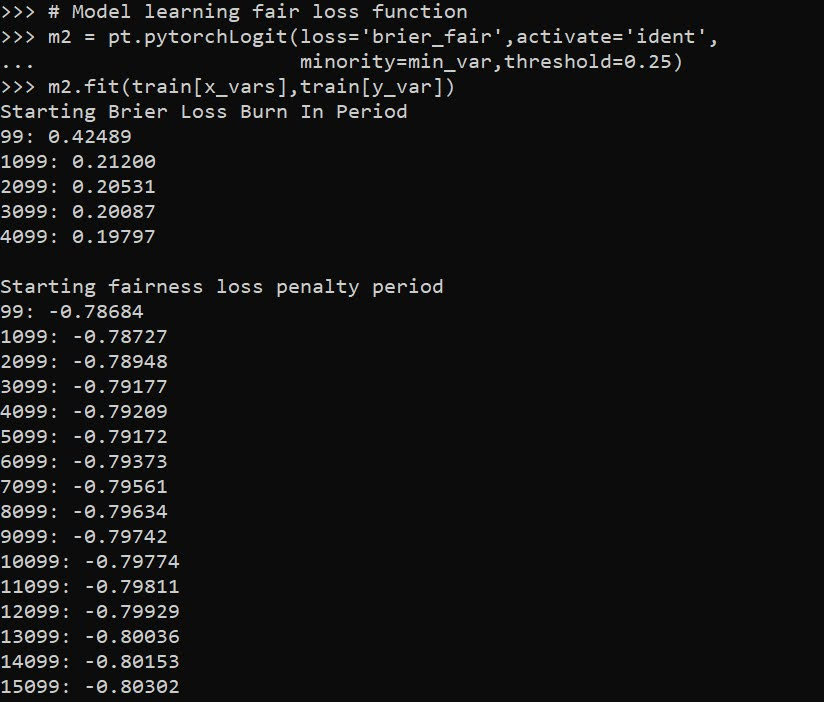I have a burn in start to get good initial parameter estimates with a more normal loss function before going into the more complicated function. Another approach would be to initialize the weights to the solution for a linear regression equation though. After that burn in though it goes into the NIJ defined fairness loss function.

Now I have functions to see how the different model metrics in whatever sample. Here you can see the model is quite balanced in terms of false positive rates in the training sample:

``````# Seeing training sample fairness
m2pp = m2.predict_proba(train[x_vars])[:,1]
ff.fairness_metric(m2pp,train[y_var],train[min_var],thresh=0.25)``````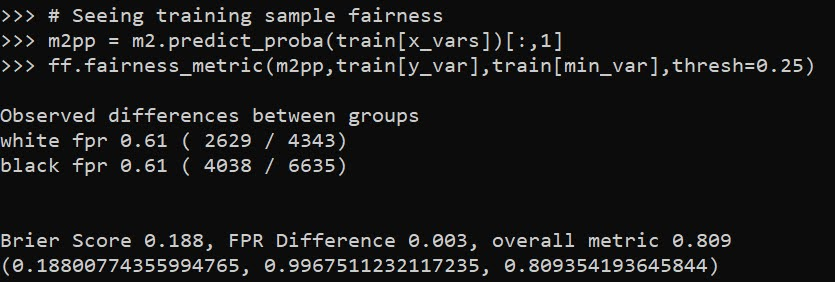But of course in the out of sample test data it is not perfectly balanced. In general you won’t be able to ensure perfect balance in whatever fairness metrics out of sample.

``````# Seeing test sample fairness
m2pp = m2.predict_proba(test[x_vars])[:,1]
ff.fairness_metric(m2pp,test[y_var],test[min_var],thresh=0.25)``````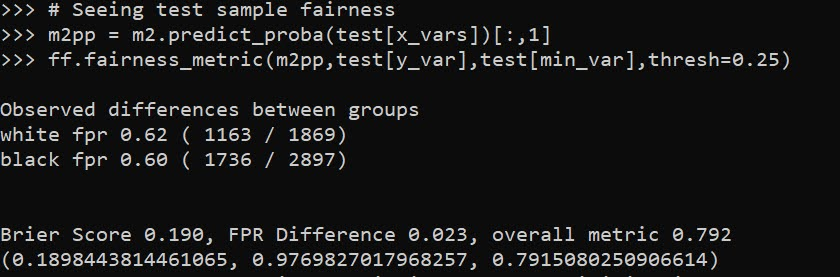It actually ends up that the difference in false positive rates between the two racial groups, even in models that do not incorporate the fairness constraint in the loss function, are quite similar. Here is a model using the same architecture but just the usual Brier Score loss. (Code not shown, see the `m1` model in the `01_AnalysisFair.py` file in the shared dropbox link earlier.)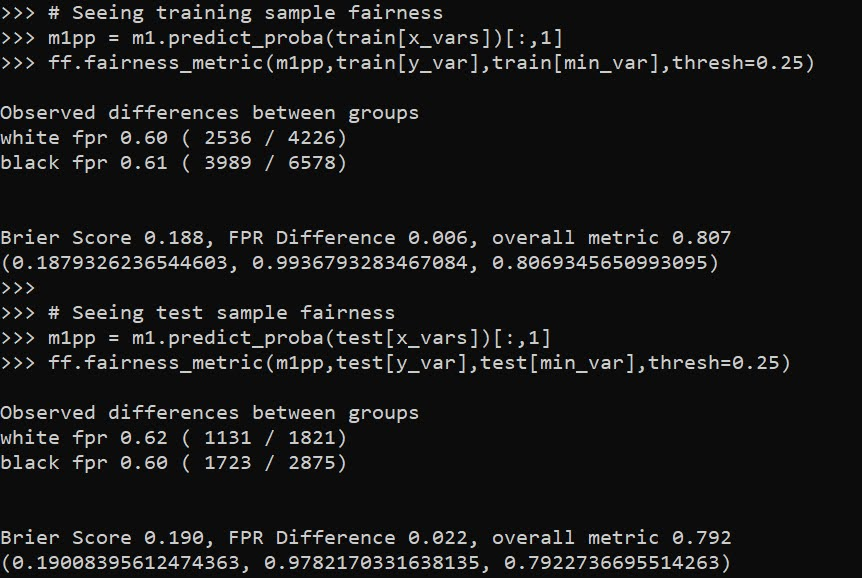You can read mine and Gio’s paper (or George Mohler and Mike Porter’s paper) about why this particular fairness function is not the greatest. In general it probably makes more sense to use an additive fairness loss instead of multiplicative, but in this dataset it won’t matter very much no matter how you slice it in terms of false positive rates. (It appears in retrospect the Compas data that started the whole false positive thing is somewhat idiosyncratic.)

There are other potential oddities that can occur with such fair loss functions. For example if you had the choice between false positive rates for `(white,black)` of `(0.62,0.60)` vs `(0.62,0.62)`, the latter is more fair, but the minority class is worse off. It may make more sense to have an error metric that sets the max false positive rate you want, and then just has weights for different groups to push them down to that set threshold.

These aren’t damning critiques of fair loss functions (these can be amended/changed to behave better), but in the end defining fair loss functions will be very tricky. Both for empirical reasons as well as for ethical ones – they will ultimately involve quite a few trade-offs.

# A latent variable approach to RTM using hidden layers in deep learning

Sorry about the long title! Previously I have blogged about how to use Deep Learning to generate an RTM like model variable selection and positive constraints. Deep learning frameworks often do not rely on variable selection like that though, they more often leverage hidden layers. For social scientists familiar with structural equation modelling, these hidden layers are very much akin to formative latent variables. (More traditionally folks use reflective latent variables in factor analysis, so the latent variable causes the observed measures. This is the obverse, the observed measures cause/define the latent variable, and we find the loadings that best predict some outcome further down the stream.)

In a nutshell, instead of the typical RTM way of picking the best variable to use, e.g. `Alcohol Density < 100 meters` OR `Alcohol Density < 500 meters`, it allows both to contribute to a latent variable, call it `AlcoholDens`, but allows those weights to vary. Then I see how well the `AlcoholDens` latent variable predicts crime. I will show later in the results that the loadings are often spread out among different density/distance measures in this sample, suggesting the approach just pick one is perhaps misguided.

I’ve posted the data and code to follow along here. There are two py files, `00_RTMHidden.py` runs the main analysis, but `dl_rtm_funcs.py` has various functions used to build the deep learning model in pytorch. I am just going to hit some of the highlights instead of walking through bit by bit.

# Some helper functions

First, last blog post I simply relied on using Poisson loss. This time, I took some effort to figure out my own loss function for the negative binomial model. Here I am using the NB2 form, and you can see I took the likelihood function from the Stata docs (they are a really great reference for various regression model info). To incorporate this into your deep learning model, you need to add a single parameter in your model, here I call it `disp`.

``````#Log likelihood taken from Stata docs, pg 11
#https://www.stata.com/manuals13/rnbreg.pdf
def nb2_loss(actual, log_pred, disp):
m = 1/disp.exp()
mu = log_pred.exp()
p = 1/(1 + disp.exp()*mu)
nll = torch.lgamma(m + actual) - torch.lgamma(actual+1) - torch.lgamma(m)
nll += m*torch.log(p) + actual*torch.log(1-p)
return -nll.mean()``````

A second set of helper functions I will illustrate at the end of the post is evaluating the fit for Poisson/Negative Binomial models. I’ve discussed these metrics before, they are just a python rewrite of older SPSS code I made.

``````def pred_nb(mu, disp, int_y):
inv_disp = 1/disp
p1 = gamma(int_y + inv_disp) / ( factorial(int_y)*gamma(inv_disp) )
p2 = ( inv_disp / (inv_disp + mu) ) ** inv_disp
p3 = ( mu / (inv_disp + mu) ) ** int_y
pfin = p1*p2*p3
return pfin

def nb_fit(mu, obs, disp, max_y):
res = []
cum_fit = mu - mu
for i in range(max_y+1):
pred_fit = pred_nb(mu=mu, disp=disp, int_y=i)
pred_obs = (obs == i)
res.append( (str(i), pred_obs.mean(), pred_fit.mean(), pred_obs.sum(), pred_fit.sum()) )
cum_fit += pred_fit
fin_fit = 1 - cum_fit
fin_obs = (obs > max_y)
res.append( (str(max_y+1)+'+', fin_obs.mean(), fin_fit.mean(),
fin_obs.sum(), fin_fit.sum()) )
dat = pd.DataFrame(res, columns=['Int','Obs','Pred','ObsN','PredN'])
return dat``````

# Main Analysis

Now onto the main analysis. Skipping the data loading (it is near copy-paste from my prior RTM Deep Learning post), here are the main guts to building and fitting the RTM model.

``````model = dl_rtm_funcs.RTM_hidden(gen_list=[alc_set,metro_set,c311_set],
gen_names=['AlcOutlets','MetroEntr','Dens311'])

for t in range(5001):
#Forward pass
y_pred = model(comb_ten)
#Loss
loss_insample = dl_rtm_funcs.nb2_loss(y_ten, y_pred, model.dispersion)
loss_insample.backward() #retain_graph=True
optimizer.step()
if t % 100 == 0:
loss_out = dl_rtm_funcs.nb2_loss(out_ten, y_pred, model.dispersion)
print(f'iter {t}: loss in = {loss_insample.item():.5f}, loss out = {loss_out.item():.5f}')``````

And in terms of iterations, on my machine this takes less than 20 seconds to do the 5000 iterations, and it has clearly peaked out by then (both in sample 2011 and out of sample 2012).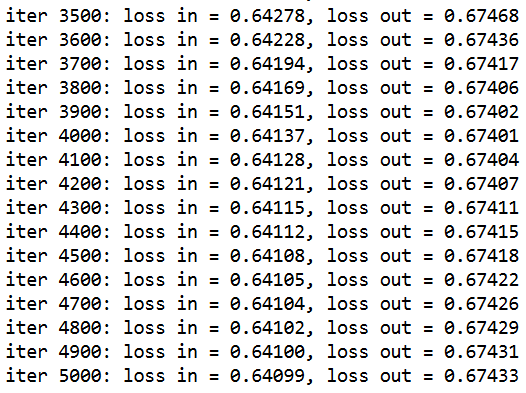I’ve loading the RTM model object with a few helper functions, so if you then run `print( model.coef_table() )`, you get out the final regression coefficients, including the dispersion term. For my negative binomial models for my dissertation, the dispersion term tended to be around ~4 for many models, so this corresponds pretty closely with my prior work.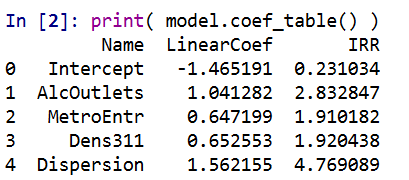These have interpretations as latent variables representing the effect of nearby alcohol outlets (both distance and density), metro entrances (just distance), and 311 calls for service (just density). Similar to original RTM, I have restricted the crime generator effects to be positive.

I also have another helper function, `model.loadings()`, that gives you a nice table. Here this shows how the original variables contribute to the latent variable. So here are the loadings for the distance to the nearest metro.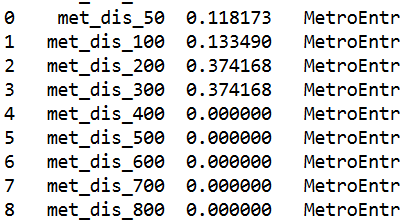You can see that the dummy variables for `met_dis_300` (meters) and smaller all contribute to the latent variable. So instead of picking one variable in the end, it allows multiple variables to contribute to the latent risk score. It may make more sense in this set up to encode variables as not cumulative, e.g. `< 50 meters, < 100 meters`, but orthogonal, e.g. `[0,50),[50,100)`, etc.), but just stuck with the prior data in the same format for now. I force the loadings to sum to 1 and be positive, so the latent variables still have a very apples-to-apples comparison in terms of effect sizes.

Here are the loadings for alcohol outlets, so we have both some distance and density effects in the end.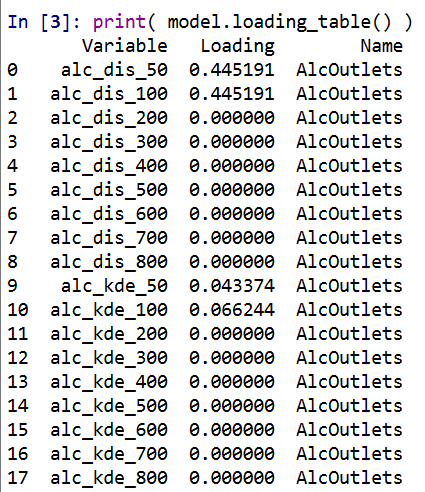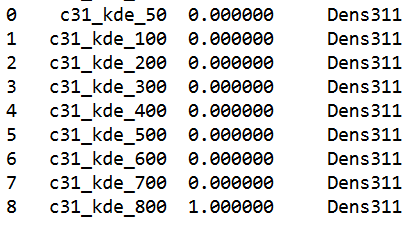So you can see for the last one, only the furthest away had an effect at all. Which is contra to the broken windows theory! But also shows that this is more general than the original RTM approach. If it only should be one variable the model will learn that, but if it should be more it will incorporate a wider array of weights.

Next is to check out how well the model does overall. For calibration for Poisson/Negative Binomial models, I just detach my pytorch tensors, and feed them into my functions to do the evaluations.

``````#Calibration for Negative Binomial predictions
pred_pd = pd.Series( y_pred.exp().detach().numpy() )
disp_val = model.dispersion.exp().item()

nb_fit = dl_rtm_funcs.nb_fit(mu=pred_pd, obs=crime_data['Viol_2011'],
disp=disp_val, max_y=10)
print( nb_fit )``````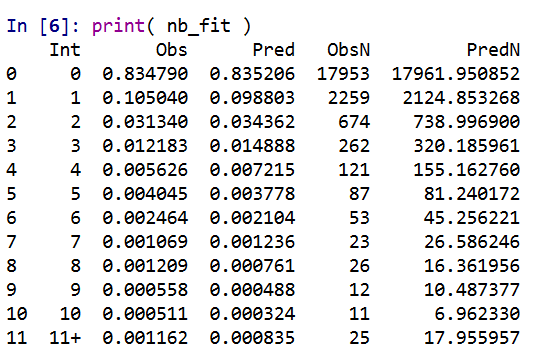So this shows that the model is pretty well calibrated in terms of overall predictions. Both samples predict 83% zeroes. I predict a few more 3/4 crime areas than observed, and my tails are somewhat thinner than they should be, but only by a tiny bit. (No doubt this would improve if I incorporated more covariates, kept it simple to debug on purpose.)

We can ignore the negative binomial dispersion term and see what our model would predict in the usual Poisson case (the mean functions are the same, it is just changing the variance). To do this, just pass in a dispersion term of 1.

``````pois_fit = dl_rtm_funcs.nb_fit(mu=pred_pd, obs=crime_data['Viol_2011'],
disp=1, max_y=10)
print( pois_fit )``````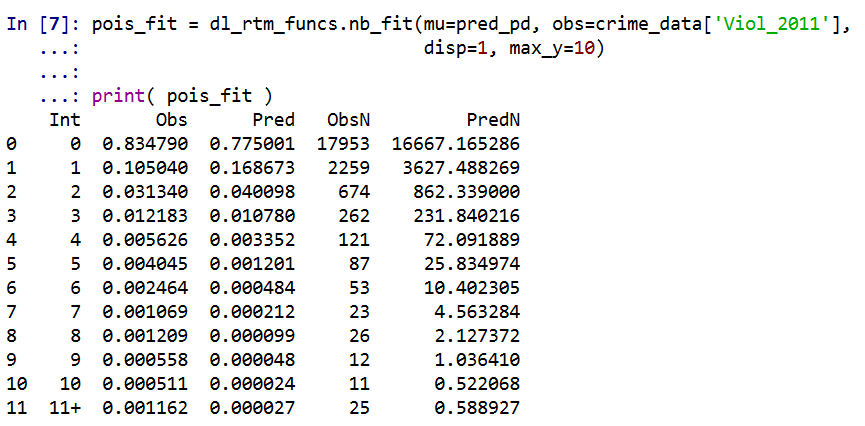You can see that the Poisson model is a much worse fit. Underpredicting zero crime areas by 6%, and areas with over 10 crimes should pretty much never happen according to the Poisson model.

We should be assessing these metrics out of sample as well, and you can see that given crime is very historically stable, the out of sample 2012 violent crime counts are similarly well calibrated.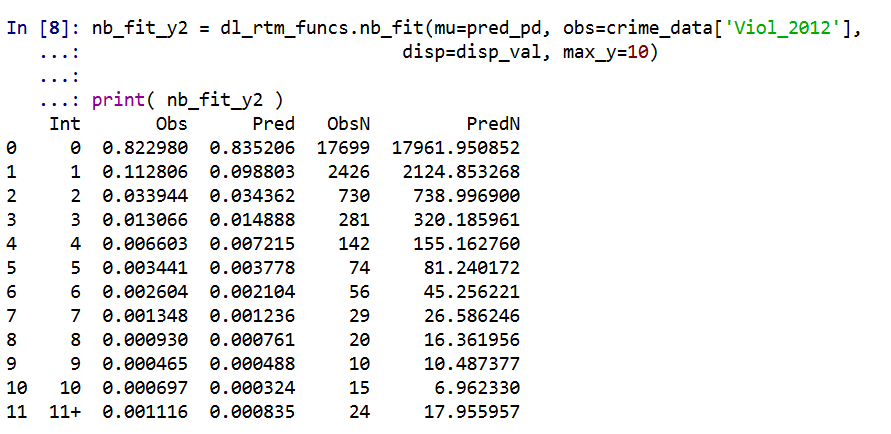Finally, I have suggested in the past to use a weighted ROC curve as a metric for crime counts. Here is a simple example of doing that in python.

``````crime_data['Weights'] = crime_data['Viol_2012'].clip(1)
crime_data['Outcome'] = crime_data['Viol_2012'].clip(0,1)

fpr, tpr, thresh = roc_curve(crime_data['Outcome'], pred_pd, sample_weight=crime_data['Weights'])
weighted_auc = auc(fpr, tpr)
print( weighted_auc ) ``````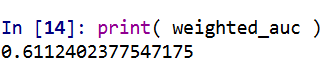So you can see the AUC is nothing to brag about here, 0.61 (it is only 0.63 in the 2011 sample). But again I am sure I could get that up by quite a bit by incorporating more covariates into the model.

# RTM Deep Learning Style

In my quest to better understand deep learning, I have attempted to replicate some basic models I am familiar with in criminology, just typical OLS and the more complicated group based trajectory models. Another example I will illustrate is doing a variant of Risk Terrain Modeling.

The typical way RTM is done is:

Data Prep Part:

1. create a set of independent variables for crime generators (e.g. bars, subway stops, liquor stores, etc.) that are either the distance to the nearest or the kernel density estimate
2. Turn these continuous estimates into dummy variables, e.g. if within 100 meters = 1, else = 0. For kernel density they typically z-score and if a z-score > 2 the dummy variable equals 1.
3. Do 2 for varying distance/bandwidth selections, e.g. 100 meters, 200 meters, etc. So you end up with a collection of distance variables, e.g. Bars_100, Bars_200, Bars_400, etc.

Modeling Part

1. Fit a Lasso regression predicting your crime outcome constraining all of the variables to be positive. (So RTM will never say a crime generator has a negative effect.)
2. For the variables that passed this Lasso stage, then do a variable selection routine. So instead of the final model having Bars_100 and Bars_400, it will only choose one of those variables.

For the modeling part, we can replicate various parts of these in a deep learning environment. For the constrain the coefficients to be positive, when you see folks refer to a “RelU” or the rectified linear unit function, all this means is that the coefficients are constrained to be positive. For the variable selection part, I needed to hack my own – it ends up being a combo of a custom dropout scheme and then pruning in deep learning lingo.

Although RTM is typically done on raster grid cells for the spatial unit of analysis, this is not a requirement. You can do all these steps on vector (e.g. street segments) or other areal spatial units of analysis.

Here I illustrate using street units (intersections and street segments) from DC. The crime generator data I take from my dissertation (and I have a few pubs in Crime & Delinquency based on that work). The crime data I illustrate using 2011 violent Part 1 UCR (homicide, agg assault, robbery, no rape in the public data).

The crime dataset is over time, and I describe in an analysis (with Billy Zakrzewski) on examining pre/post crime around DC medical marijuana dispensaries.

The data and code to replicate can be downloaded here. It is python, and for the deep learning model I used pytorch.

# RTM Example in Python

So I will walk through briefly my second script, `01_DeepLearningRTM.py`. The first script, `00_DataPrep.py`, does the data prep, so this data file already has the crime generator variables prepared in the manner RTM typically creates them. (The `rtm_dl_funcs.py` has the functions to do the feature extraction and create the deep learning model, to do distance/density in sci-kit is very slick, only a few lines of code.)

So first I just define the libraries I will be using, and import my custom rtm functions I created.

``````######################################################
import numpy as np
import pandas as pd
import torch
device = torch.device("cuda:0")
import os
import sys

my_dir = r'C:\Users\andre\OneDrive\Desktop\RTM_DeepLearning'
os.chdir(my_dir)
sys.path.append(my_dir)
import rtm_dl_funcs
######################################################``````

The next set of code grabs the crime data, and then defines my variable sets. I have plenty more crime generator data from my dissertation, but to make it easier on myself I just focus on distance to metro entrances, the density of 311 calls (a measure of disorder), and the distance and density of alcohol outlets (this includes bars/liquor stores/gas stations that sell beer, etc.).

Among these variable sets, the final selected model will only choose one within each set. But I have also included the ability for the model to incorporate other variables that will just enter in no-matter what (and are not constrained to be positive). This is mostly to incorporate an intercept into the regression equation, but here I also include the percent of sidewalk encompassing one of my street units (based on the Voronoi tessellation), and a dummy variable for whether the street unit is an intersection. (I also planned on included the area of the tessalation, but it ended up being an explosive effect, my dissertation shows its effect is highly non-linear, so didn’t want to worry about splines here for simplicity.)

``````######################################################
#Get the Prepped Data

#Variable sets for each
db = [50, 100, 200, 300, 400, 500, 600, 700, 800]
metro_set = ['met_dis_' + str(i) for i in db]
alc_set = ['alc_dis_' + str(i) for i in db]
alc_set += ['alc_kde_' + str(i) for i in db]
c311_set = ['c31_kde_' + str(i) for i in db]

#Creating a few other generic variables
crime_data['PercSidewalk'] = crime_data['SidewalkArea'] / crime_data['AreaMinWat']
crime_data['Const'] = 1
const_li = ['Const','Intersection','PercSidewalk']
full_set = const_li + alc_set + metro_set + c311_set
######################################################``````

The next set of code turns my data into a set of torch tensors, then I grab the size of my independent variable sets, which I will end up needing when initializing my pytorch model.

Then I set the seed (to be able to reproduce the results), create the model, and set the loss function and optimizer. I use a Poisson loss function (will need to figure out negative binomial another day).

``````######################################################
#Now creating the torch tensors
x_ten = torch.tensor(crime_data[full_set].to_numpy(), dtype=float)
y_ten = torch.tensor(crime_data['Viol_2011'].to_numpy(), dtype=float)
out_ten = torch.tensor(crime_data['Viol_2012'].to_numpy(), dtype=float)

#These I need to initialize the deep learning model
gen_lens = [len(alc_set), len(metro_set), len(c311_set)]

#Creating the model
torch.manual_seed(10)

model = rtm_dl_funcs.RTM_torch(const=len(const_li),
gen_list=gen_lens)
criterion = torch.nn.PoissonNLLLoss(log_input=True, reduction='mean')
print( model )
######################################################``````

If you look at the printed out model, it gives a nice summary of the different layers. We have our one layer for the fixed coefficients, and another three sets for our alcohol outlets, 311 calls, and metro entrances. We then have a final cancel layer. The idea behind the final cancel layer is that the variable selection routine in RTM can still end up not selecting any variables for a set. I ended up not using it here though, as it was too aggressive in this example. (So will need to tinker with that some more!)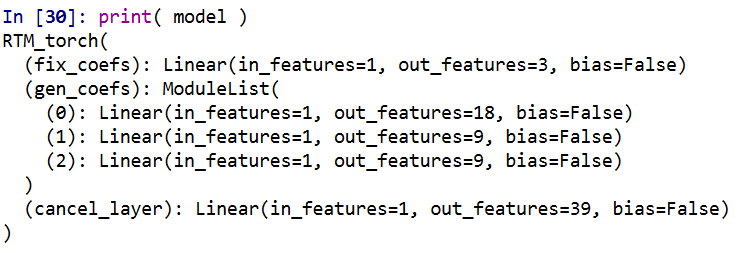The variable selection routine is very volatile – so if you have very correlated inputs, you can essentially swap one for the other and get near equivalent predictions. I often see folks who do RTM analyses say something along the lines of, “OK this RTM selected A, and this RTM selected B, so they are different effects in these two samples” (sometimes pre/post, other times comparing different areas, and other times different crime outcomes). I think this is probably wrong though to make that inference, as there is quite a bit of noise in the variable selection process (and the variable selection process itself precludes making inferences on the coefficients themselves).

My deep learning example inherited the same problems. So if you change the initialized weights, it may end up selecting totally different inputs in the end. To get the variable selection routine to at least select the same crime generator variables in my tests, I do a burn in period in which I implement a random dropout scheme. So instead of the typical dropout, for every forward pass it does a random dropout to only select one variable randomly out of each crime generator set. After that converges, I then use a pruning layer to only pick the coefficient that has the largest effect, and again do a large set of iterations to make sure the results converged. So different means but same ends to the typical RTM steps 4 and 5 above. I also have like I said a ReLU transformation after each layer, so the crime generator variables are always positive, any negative effects will be pruned out.

One thing that is nice about deep learning is that it can be quite fast. Here each of these 10,000 iteration sets take less than a minute on my desktop with a GPU. (I’ve been prototyping models with more parameters and more observations at work on my laptop with just a CPU that only take like 10 to 20 minutes).

``````######################################################
#Burn in part, random dropout
for t in range(10000):
#Forward pass
y_pred = model(x=x_ten)
#Loss
loss_insample = criterion(y_pred, y_ten)
loss_insample.backward(retain_graph=True)
optimizer.step()
if t % 1000 == 0:
print(f'loss: {loss_insample.item()}' )

#Switching to pruning all but the largest effects
model.l1_prune()

for t in range(10000):
#Forward pass
#Loss
loss_insample = criterion(y_pred, y_ten)
loss_insample.backward(retain_graph=True)
optimizer.step()
if t % 1000 == 0:
print(f'loss: {loss_insample.item()}' )

print( model.coef_df(nm_li=full_set, cancel=False) )
######################################################``````

And this prints out the results (as incident rate ratios), so you can see it selected 50 meters alcohol kernel density, 50 meters distance to the nearest metro station, and kernel density for 311 calls with an 800 meter bandwidth.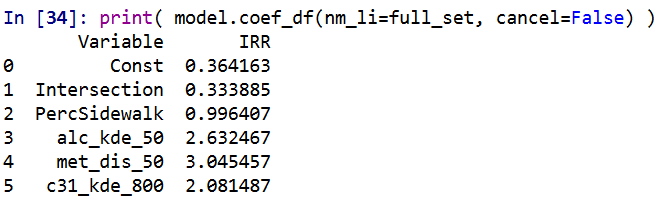I have in the code another example model when using a different seed. So testing out on around 5 different seeds it always selected these same distance/density variables, but the coefficients are slightly different each time. Here is an example from setting the seed to 12.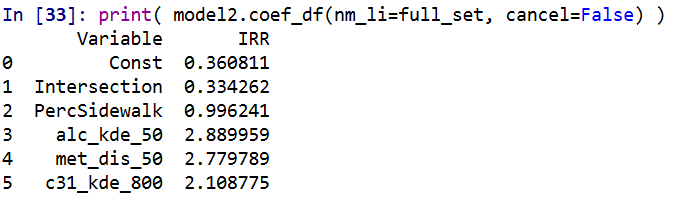These models are nothing to brag about, using the typical z-score the predictions and set the threshold to above 2, the PAI is only around 3 (both for in-sample 2011 and out of sample 2012 is slightly lower). It is a tough prediction task – the mean number of violent crimes per street unit per year is only 0.3. Violent crime is fortunately very rare!

But with only three different risk variables, we can do a quick conjunctive analysis, and look at the areas of overlap.

``````######################################################
#Adding model 1 predictions back into the dataset
crime_data['Pred_M1'] = pred_mod1

#Check out the areas of overlapping risk
mod1_coef = model.coef_df(nm_li=full_set, cancel=False)
risk_vars = list(set(mod1_coef['Variable']) - set(const_li))
conj_set = crime_data.groupby(risk_vars, as_index=False)['Const','Pred_M1','Viol_2012'].sum()
print(conj_set)
######################################################``````

In this table `Const` is the total number of street units selected, `Pred_M1` is the expected number of crimes via Model 1, and then I show how well it conforms to the predictions out of sample 2012. So you can see in the aggregate the predictions are not too far off. There only ends up being one street unit that overlaps for all three risk factors in the study area.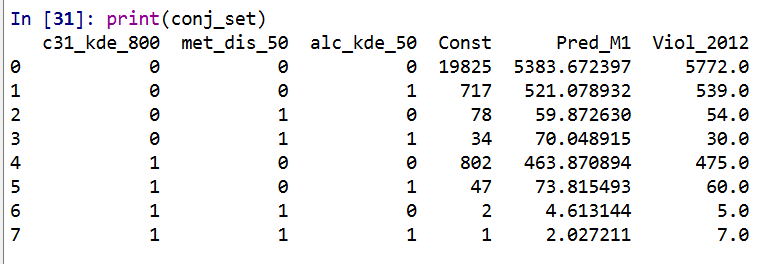I believe the predictions would be better if I included more crime generator variables. But ultimately the nature of how RTM works it trades off accuracy for simple models. Which is fair – it helps to ease the nature of how a police department (or some other entity) responds to the predictions.

But this trade off results in predictions that don’t fare as well compared with more complicated models. For example I show (with Wouter Steenbeek) that random forests do much better than RTM. To make those models more interpretable we did local decompositions for hot spots, so say this hot spot is 30% alcohol outlets, 20% nearby apartments, etc.

So there is no doubt more extensions for RTM you could do in a deep learning framework, but they will likely always result in more complicated and less interpretable models. Also here I don’t think this code will be better than the traditional RTM folks, the only major benefit of this code is it will run faster – minutes instead of overnight for most jobs.

# GPU go brrr: Estimating OLS (with standard errors) via deep learning

So a bunch of my criminologists friends have methods envy. So to help them out, I made some python functions to estimate OLS models using pytorch (a deep learning python library). So if you use my functions, you can just append something like an analysis with GPU accelerated deep learning to the title of your paper and you are good to go. So for example, if your paper is `An analysis of the effects of self control on asking non-questions at an ASC conference`, you can simply change it to `A GPU accelerated deep learning analysis of the effects of self control on asking non-questions at an ASC conference`. See how that works, instantly better.

Code and data saved here. There are quite a few tutorials on doing OLS in deep learning libraries, only thing special here is I also calculate the standard errors for OLS in the code as well.

# python code walkthrough

So first I just import the libraries I need. Then change the directory to wherever you saved the code on your local machine, and then import my `deep_ols` script. The `deep_ols` script also relies on the `scipy.stats` library, but besides pytorch it is the usual scientific python stack.

``````import os
import sys
import torch
import statsmodels.api as sm
import pandas as pd
import numpy as np

###########################################
#Setting the directory and importing
#My deep learning OLS function

my_dir = r'C:\Users\andre\OneDrive\Desktop\DeepLearning_OLS'
os.chdir(my_dir)
sys.path.append(my_dir)
import deep_ols
############################################``````

For the dataset I am using, it is a set of the number of doctor visits I took from some of the Stata docs.

``````#Data from Stata, https://www.stata-press.com/data/r16/gsem_mixture.dta
#see pg 501 https://www.stata.com/manuals/sem.pdf

visit_dat['intercept'] = 1
y_dat = visit_dat['drvisits']
x_dat = visit_dat[['intercept','private','medicaid','age','educ','actlim','chronic']]

print( y_dat.describe() )
print( x_dat.describe() )``````

Then to estimate the model it is simply below, the function returns the torch model object, the variance/covariance matrix of the coefficients (as a torch tensor), and then a nice pandas data frame of the results.

``mod, vc, res = deep_ols.ols_deep(y=y_dat,x=x_dat)``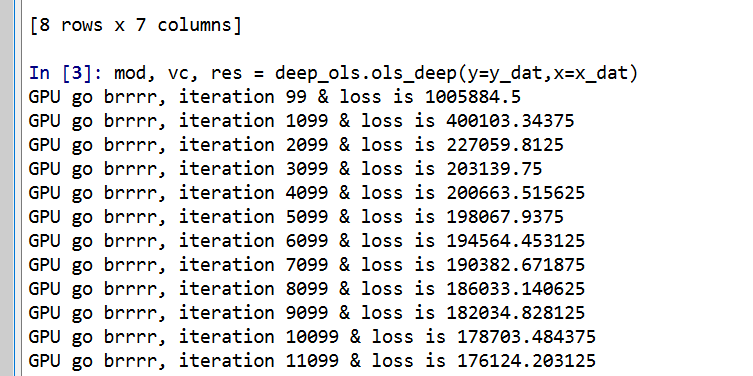As you can see, it prints out GPU accelerated loss results so fast for your pleasure.

Then, like a champ you can see the OLS results. P-values < 0.05 get a strong arm, those greater than this get a shruggie. No betas to be found in my model results.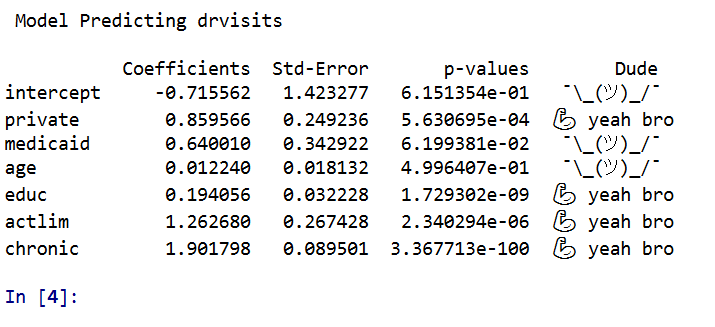Just to confirm, I show you get the same results using `statsmodel`.

``````stats_mod = sm.OLS(y_dat,x_dat)
sm_results = stats_mod.fit()
print(sm_results.summary())``````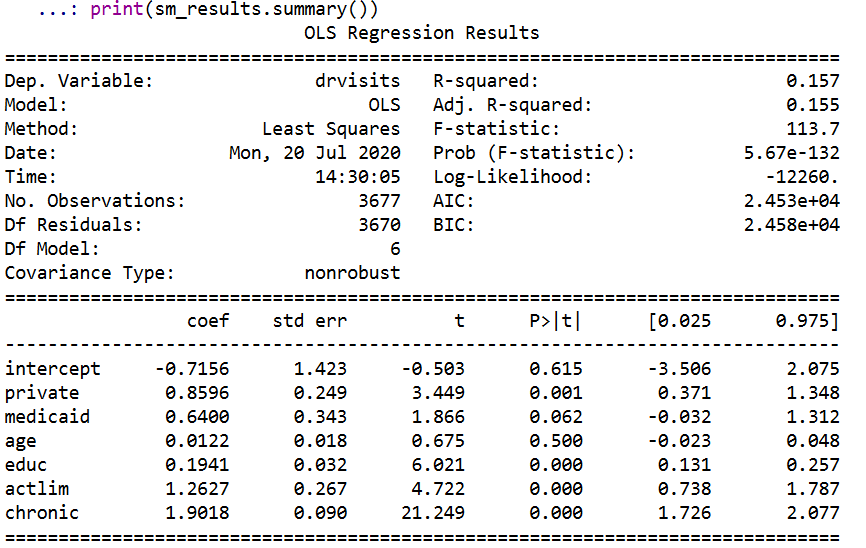So get on my level bro and start estimating your OLS models with a GPU.

# Some Notes

First, don’t actually use this in real life. I don’t do any pre-processing of the data, so if you have some data that is not on a reasonable scale (e.g. if you had a variable income going from 0 to \$500,000) all sorts of bad things can happen. Second, it is not really accelerated – I mean it is on the GPU and the GPU goes brr, but I’m pretty sure this will not ever be faster than typical OLS libraries. Third, this isn’t really “deep learning” – I don’t have any latent layers in the model.

Also note the standard errors for the coefficients are just calculated using a closed form solution that I pilfered from this reddit post. (If anyone has a textbook reference for that would appreciate it! I Maria Kondo’d most of my text books when I moved out of my UTD office.) If I figure out the right gradient calculations, I could probably also add ‘robust’ somewhere to my suggested title (by using the outer product gradient approach to get a robust variance/covariance matrix for the coefficients).

As a bonus in the data file, I have a python script that shows how layers in a vanilla deep learning model (with two layers) are synonymous with a particular partial least squares model. The ‘relu activation function’ is equivalent to a constraint that restricts the coefficients to be positive, but otherwise will produce equivalent predictions.

You could probably do some nonsense of saving the Jacobian matrix to get standard errors for the latent layers in the neural network if you really wanted to.

To end, for those who are really interested in deep learning models, I think a better analogy is that they are just a way to specify and estimate a set of simultaneous equations. Understanding the nature of those equations will help you relate how deep learning is similar to regression equations you are more familiar with. The neuron analogy is just nonsense IMO and should be retired.

# Using pytorch to estimate group based traj models

Deep learning, tensors, pytorch. Now that I have that seo junk out of the way 🙂 – I’ve been trying to teach myself some “Deep Learning”, as it is what all of the cool kids are doing these days.

I was having a hard time though with many of the different examples. Many are for image data, and so it was hard for me to translate that to actual applications I am interested in. Many do talk about dimension reduction and reducing to hidden layers, so I thought that was similar in nature to latent class analysis, such as group-based-trajectory-modelling (GBTM).

If you aren’t familiar with GBTM, imagine a scenario in which you cluster data, and then you estimate a different regression model to predict some outcome for each subset of the clustered data. This is just a way to do that whole set-up in one go, instead of doing each part separately. It has quite a few different names – latent class analysis and mixture modelling are two common ones. The only thing really different about GBTM is that you have repeated observations – so if you follow the same person over time, they should always be assigned to the same cluster/mixture.

In short you totally can do GBTM models in deep learning libraries (as I will show), but actually most examples that I have walked through are more akin to dimension reduction of columns (so like PCA/Canonical Correlation). But the deep learning libraries are flexible enough to do the latent class analysis I want here. As far as I can tell they are basically just a nice way to estimate systems of equations (with a ton of potential parameters, and do it on the GPU if you want).

So I took it as a challenge to estimate GBTM models in a deep learning library – here pytorch. In terms of the different architectures/libraries (e.g. pytorch, tensorflow, Vowpal Wabbit) I just chose pytorch because one of my co-workers suggested pytorch was easier to learn!

I’ve posted a more detailed notebook of the code, but it worked out quite well. So first I simulated two groups of data (50 observations in each group and 11 time periods). I added a tiny bit of random noise, so this (I was hoping) should be a pretty tame problem for the machine to learn.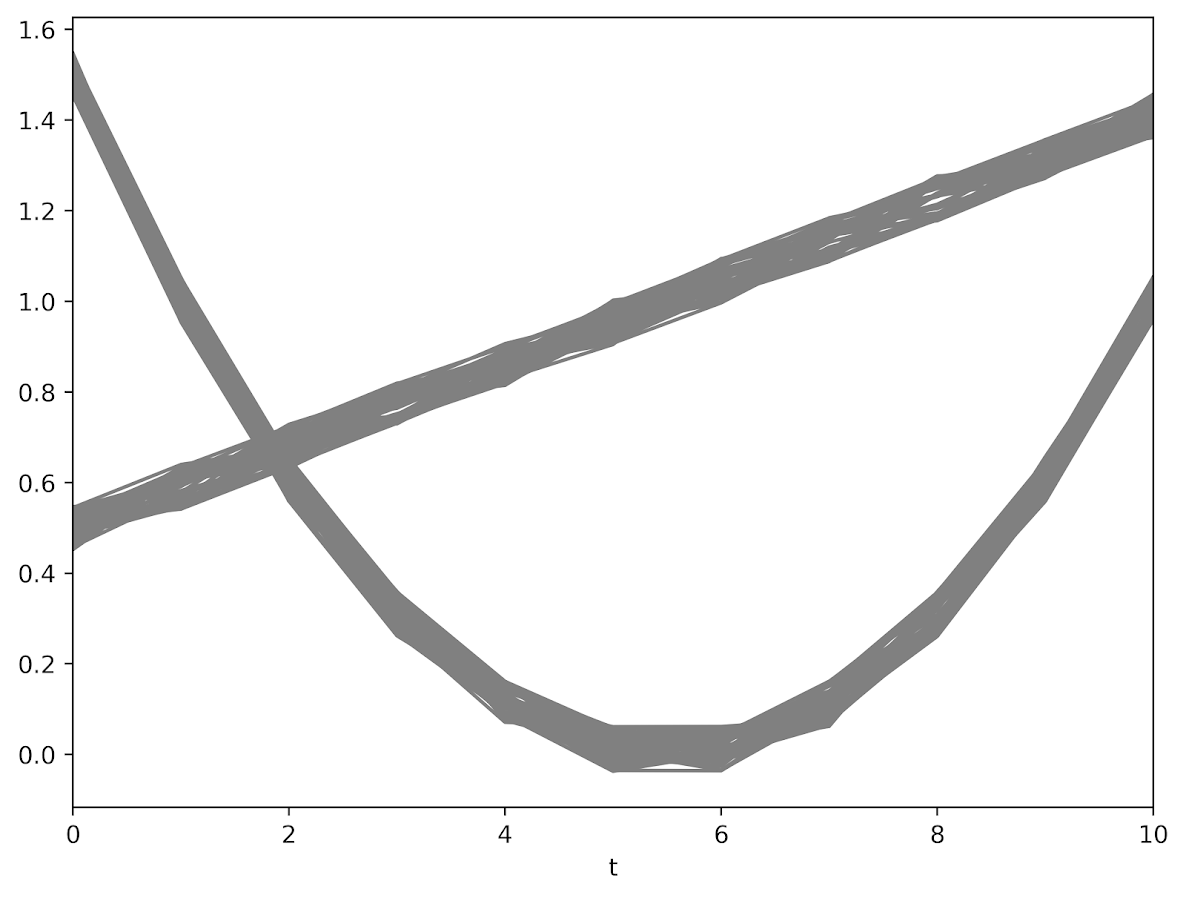The code to generate a pytorch module and have the machine churn out the gradients is pretty slick (less than 30 lines total of non-comments). Many GBTM code bases make you do the analysis in wide format (so one row is an observation), but here I was able to figure out how to set it up in long data format, which makes it real easy to generalize to unbalanced data.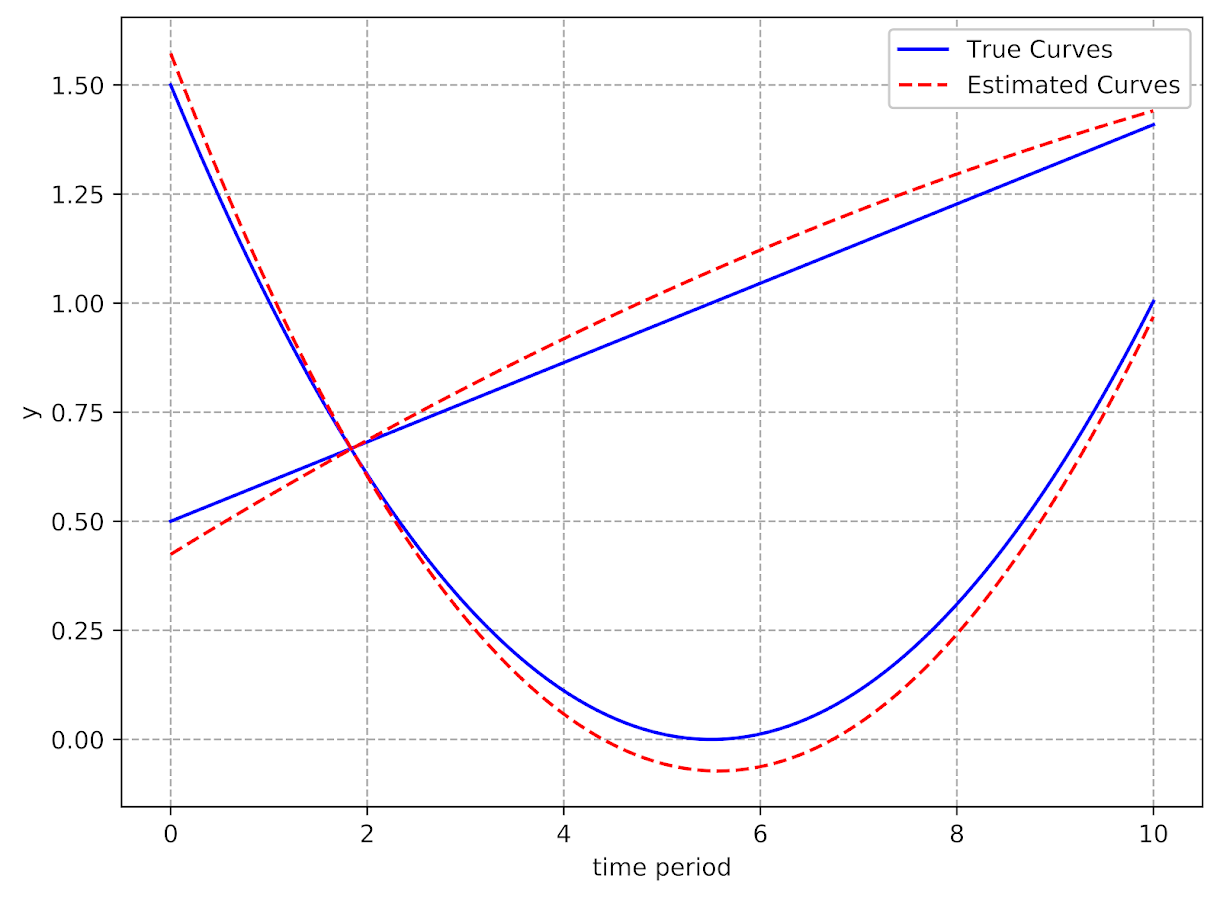It took quite a few iterations to converge though, (iterations were super fast, but it is a tiny problem, so not sure how timing will generalize) and only converged when using the Adam optimizer (stochastic gradient descent converged to an answer with a similar mean square error, but not to anywhere near the right answer). These models are notorious for converging to sub-optimal locations, so that may just be an intrinsic part of the problem and a good library needs to do better with starting conditions.

I have a few notes about potential updates to the code at the end of my Jupyter notebook. For count or binomial 0/1 data, that should be a pretty easy update. Also need to write code to do out of sample predictions (which I think I can figure out as well). A harder problem I am not sure how to figure out is to do an equation for the latent groups inside of the function. And I don’t know how to get standard errors for the coefficient estimates. Hopefully I can figure that out while trying to teach myself some more deep learning. I have a few convolution ideas I want to try out for spatial-temporal crime forecasting and include proactive police feedback, but I won’t get around to them for quite awhile I imagine.